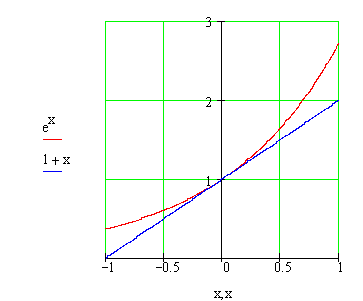Calculus on Demand at Dartmouth College Lecture 12 | Index | Lecture 14 Lecture 13Resources

Math 3 Course Syllabus
Practice Exams

Contents

In this lecture we develop formulas for the derivatives of exponential and logarithmic functions.

Quick Question

What is the slope of the graph of the function ex at x = 0?Outline

Outline for Derivatives of Exponential and Logarithm Functions

Textbook

Derivatives of Exponential and Logarithm Functions